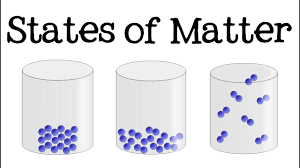# States of Matter: Different states and Their Properties

In this quiz, you will find questions related to the different states of matter (solid, liquid, and gas) and their properties.Start Quiz

Which energy is generated from due to the motion of its particles?

Thermal Muscular Momentary Potential

Which of the following posses highest thermal energy?

Water at 0° Iron at 37° Milk at 50° Chocolate at 26°

Thermal energy is an example of __________

kinetic energy potential energy muscular energy momentary energy

The faster the body moves, the higher the thermal energy.

True False

___________ of particles results in the rise of thermal energy.

Direction Vibration Shape Atoms

A container with a pin-hole contains equal moles of H2(g) and O2(g). Find the fraction of oxygen gas escaped at the same time when one-fourth of hydrogen gas escapes

1/16 1/4 1/2 1/8

If the temperature is doubled, the average velocity of a gaseous molecule increases by

4 1.4 2 2.8

Find the molecular mass of a gas that takes three times more time to effuse as compared to He with the same volume

9 u 64 u 27 u 36 u

At the same temperature, the average molar kinetic energy of N2 and CO is

KE1 > KE2 KE1 < KE2 KE1 = KE2 insufficient information given

The rate of diffusion methane is twice that of X. The molecular mass of X is

64.0 32.0 40 80

Name the liquid with higher vapour pressure in the following pairs:

Alcohol, glycerine Petrol, kerosene mercury, water.(a) Alcohol, Water, Petrol(b) Petrol, Water, Alcohol(c) Alcohol, Petrol, Water None of these

The state of matter that shows the uniformity of behavior :

Solid Liquid Liquid Gas None of the Above

A gas deviates from ideal behavior at a high pressure because its molecules:

Attract one another Show the Tyndall Effect Have kinetic energy Are bound by covalent bonds

The value of universal gas constant R depends on

Temperature of Gas Volume of Gas Number of Moles of Gas Units of Volume,Temperature and Pressure

What are the conditions for gas like Carbon monoxide to obey the ideal gas laws?

Low temperature and low pressure

Low temperature and high pressure

High temperature and low pressure

High temperature and high pressure

Who proposed the concept of dispersion force ?

Fritz London van der Waal Heitler and London Gay Lussac

Dipole-dipole interaction is stronger than the London forces but is weaker than ion-ion interaction because

Only partial charges are involved

Only total charges are involved

Both

None of these

Which of the following is not a type of Vander Waal’s forces?

Ion - dipole forces

London forces

Dipole - induced dipole forces

Dipole - dipole forces

The interaction energy of London force is inversely proportional to sixth power of the distance between two interacting particles but their magnitude depends upon

Polarisability of interacting particles

Strength of permanent dipoles in the particles

Mass of interacting particles

Charge of interacting particles

Dipole-dipole forces act between the molecules possessing permanent dipole. Ends of dipoles possess ‘partial charges’. The partial charge is

Less than unit electronic charge

Double the unit electronic charge

Equal to unit electronic charge

More than unit electronic charge

Quiz/Test Summary
Title: States of Matter: Different states and Their Properties
Questions: 20
Contributed by: